Next: Algorithm of event generation Up: Beamstrahlung Previous: Beamstrahlung

### Basic formulas

When the orbit of a high energy electron(positron) with energy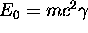is bent by a magnetic field B or by an electric field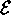with the curvature radius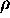, the critical energy of synchrotron radiation is given by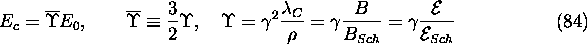where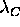is the Compton wavelength,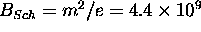Tesla is Schwinger's critical field  and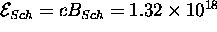V/m.

The energy spectrum of emitted photons is given by the Sokolov-Ternov formula . (Radiation angle is not included in CAIN. All the photons are emitted forward.) The number of photons per unit time in the interval (x,x+dx) of the energy fraction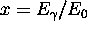is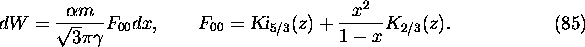Here,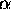is the fine structure constant and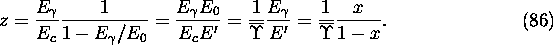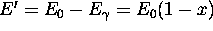is the final electron energy,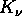the modified Bessel function and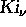is its integral: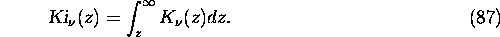Toshiaki Tauchi
Thu Dec 3 17:27:26 JST 1998